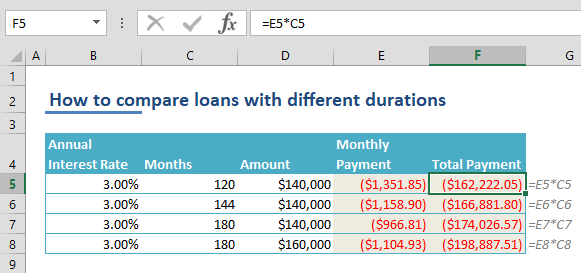Want to get a loan for a home or vehicle, but can't decide which option is the best? Use Excel to compare your options and make better decisions! Excel's PMT function is perfect for this type of analyses. In this article, we're going to show you how to compare loans with different durations in Excel using the PMT function.

## Calculating the payments of a loan

The PMT function can calculate the payments of a loan with a given constant interest rate. The function assumes that the debtor pays constant amounts for each repayment interval.

The PMT function can take 5 arguments. While 3 of them are required, the remaining 2 are optional arguments. You need to supply the interest rate (rate), number of payment periods (nper), and the loan value (pv). If you want to also incorporate a remaining value, you can also enter that number for the fv argument. Finally, the type argument determines when the repayments are due. The default option is at the end of each period.

Syntax:

PMT(rate, nper, pv, [fv], [type])

Here is how you can calculate a payment amount for a \$140,000 loan with constant payments over 120 months, with a 3.00% annual interest rate.

=PMT(0.03/12,120,140000)As shown in the example above, the annual interest rate is divided by 12 to match the actual rate to the period used in the formula (months). If we use 10 years instead of 120 month, we could use the 3.00% annual rate without dividing it into months.

## Comparing loans with different durations

Finding the payment amount is as simple as using plugging in your numbers into a single formula. Our next step is to add other loan options as new rows, and create a table.This structure makes the comparison much easier. Feel free to run your analysis by changing any of the variables. For example, the loan option on the 4th row has a loan amount 160,000.

To make this table easier to read, we can add a column to show the total payment amount at the end of all payment periods. To do this, we just need to add a formula to calculate months * monthly payment.That's all! You can now easily compare different loans side-by-side.